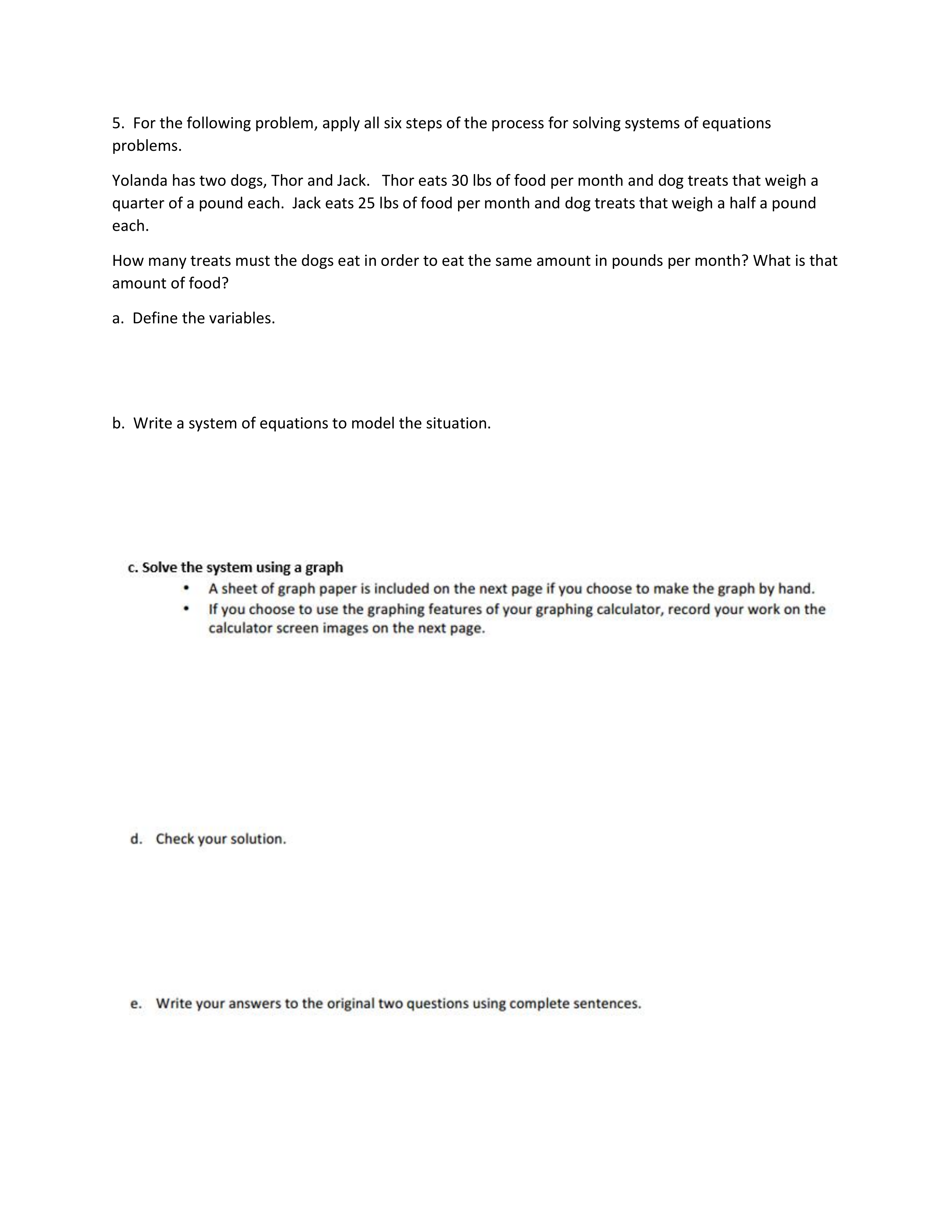Reviewing Systems
starstarstarstarstarstarstarstarstarstar
by Kyle Marks
| 10 Questions
1
5 pts
Yolanda has two dogs, Thor and Jack. Thor eats 30 lbs of food per month and dog treats that weigh a quarter of a pound each. Jack eats 25 lbs of food per month and dog treats that weigh a half a pound each.
How many treats must the dogs eat in order to eat the same amount in pounds per month? What is that amount of food? (Note: number 5 might be a helpful place to start with this problem)
2
5 pts
The product of two numbers of 48. The difference of those numbers is 2.
What are the two numbers? Define your variables. Write a system of equations.3
3
5 pts
Order the list to show how you would solve this system of inequalities.
1. connect your points with a dashed line
2. plot a point at 9 on the y axis
3. plot a point at -9 on the y axis
4. use the slope 5/2 and continue to plot points going up five to the right two
5. connect your points with a solid line
6. use the slope -2 and continue to plot points going down two to the right one
7. use a point like (0,0) to determine where to shade for each inequality
8. shade the area where both shaded areas would overlap
4
5 pts
Solve the system of equations by graphing by hand. report your solution and check that your solution is correct.5
5
5 pts
Define the variables in this question. (Note: this is expanding from question 1)
Let T be the number of treats Thor and Jack eat
Let T be the pounds of food that Thor eats
Let F be the total pounds of food and treats that Thor and Jack eat
Let J be the pounds of food that Jack eats6
6
10 pts
Write the system of equations for this situation. Use capital letters for your variables and no spaces in the equations. use one space to separate the equations.7
7
5 pts
In words explain the meaning of the solution.

8
5 pts
After watching the video, graph the system of inequalities on this graph.
9
10 pts
Match the statements to the correct inequality symbols.
• less than
• less than or equal to
• greater than
• greater than or equal to
• not equal to
• is smaller than
• no more than
• more than
• at least
• cannot equal
• below
• maximum
• is larger than
• no less than
• is not the same
• is under
• at most
• above
• minimum
• does not exceed
• exceeds
• <
• >
Add to my formatives list

Formative uses cookies to allow us to better understand how the site is used. By continuing to use this site, you consent to the Terms of Service and Privacy Policy.